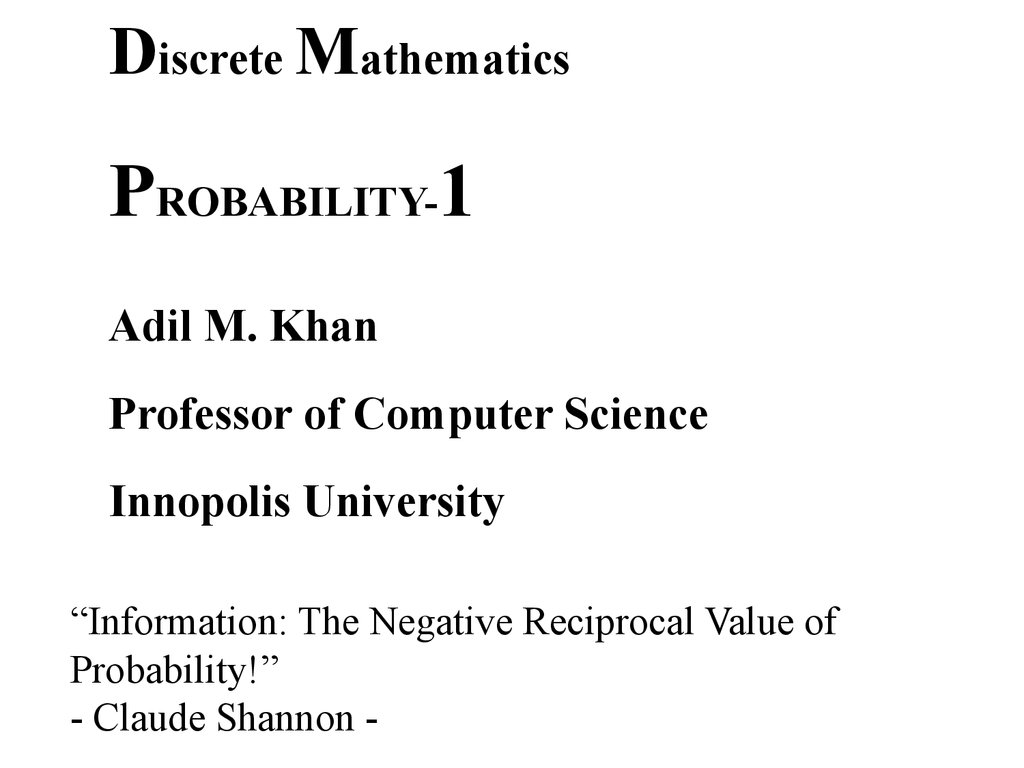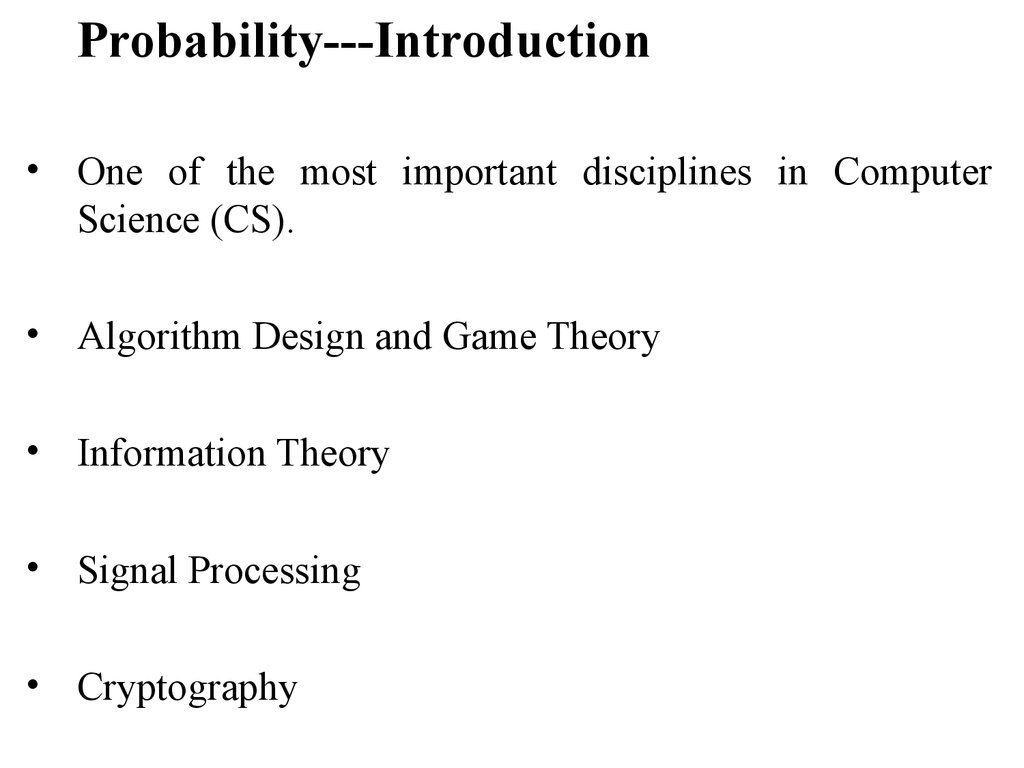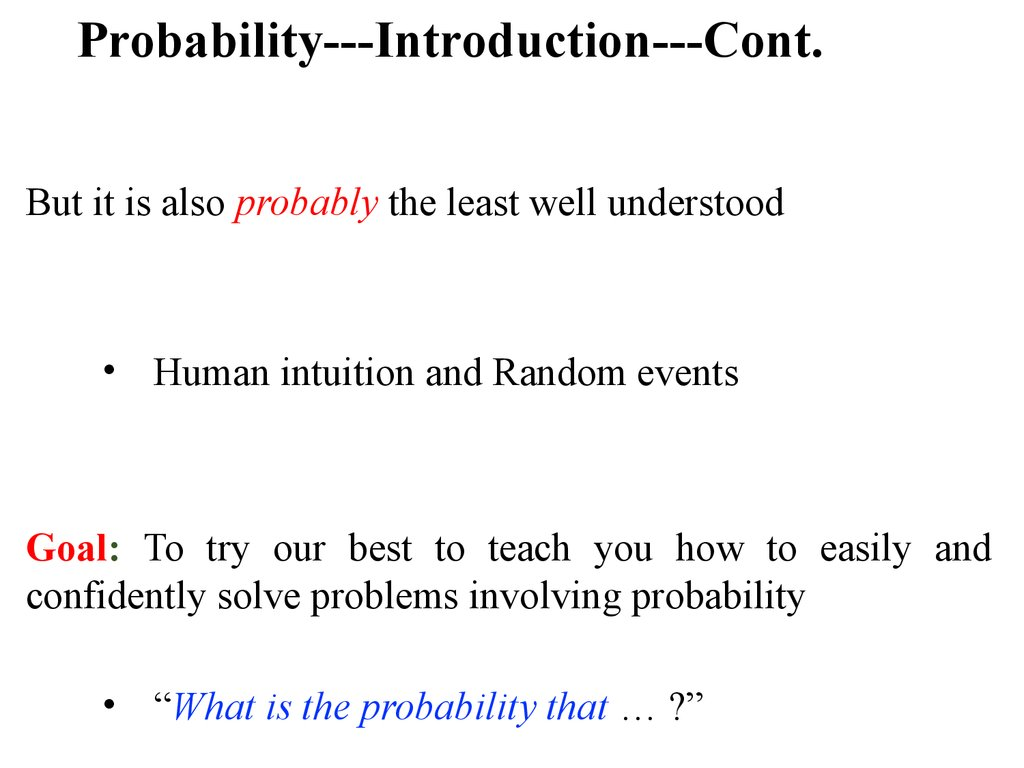Discrete mathematics. Probability

1.

Discrete Mathematics
PROBABILITY-1
Professor of Computer Science
Innopolis University
“Information: The Negative Reciprocal Value of
Probability!”
- Claude Shannon -

2.

Probability---Introduction
• One of the most important disciplines in Computer
Science (CS).
• Algorithm Design and Game Theory
• Information Theory
• Signal Processing
• Cryptography

3.

Probability---Introduction---Cont.
But it is also probably the least well understood
• Human intuition and Random events
Goal: To try our best to teach you how to easily and
confidently solve problems involving probability
• “What is the probability that … ?”

4.

Probability
Contents
• Basic definitions and an elementary 4-step process
• Counting
• Conditional probability and the concept
independence
• Random Variable
• Expected value and Standard Deviation
of

5.

Probability
Let’s Make a Deal
• The famous game show (you might have seen this
• Participant is given a choice of three doors. Behind one
door is a car, behind the others, useless stuff. The
participant picks a door (say door 1). The host, who
knows what is behind the doors, opens another door (say
door 3) which has the useless stuff. He then asks the
participant if he would like to switch (pick door 2)?
Is it to participant’s advantage to switch or not?

6.

Probability
Precise Description
• The car is equally likely to be hidden behind the three
doors.
Equally likely events are events that have the same
likelihood of occurring. For example. each numeral on a die is
equally likely to occur when the die is tossed.

7.

Probability
Precise Description
• The car is equally likely to be hidden behind the three
doors.
• The player is equally likely to pick each of the doors.
• After the player picks a door, the host must open a
different door (with the useless thing behind it) and
offer the player to switch.
When a host has a choice of which door to pick, he is
equally likely to pick each of them.
Now here comes the question:
“What is the probability that a player who switches wins the

8.

Probability
Solving Problems Involving Probability
Model the situation mathematically
Solve the resulting mathematical problem

9.

Probability
Solving Problems Involving Probability
Step 1: Finding the sample space
Set of all possible outcomes of a random process
To say that a process is random means that when it takes
place, one outcome from a set of outcomes is sure to occur,
but it is impossible to predict with certainty which outcome
that will be.
For example: tossing a coin, choosing winners in state lotteries.

10.

Probability
Solving Problems Involving Probability
Step 1: Finding the sample space
• Set of all possible outcomes of a random process
The set of all possible outcomes that can result from a random
process is is called a sample
space.

11.

Probability
Solving Problems Involving Probability
Step 1: Finding the sample space
• Set of all possible outcomes of a random process
To find this, we must understand the quantities involve in
the random process

12.

Probability
Solving Problems Involving Probability
Step 1: Finding the sample space
• Set of all possible outcomes of a random process
To find this, we must understand the quantities involve in
the random process
Quantities in the above problem:
• The door concealing the car
• The door initially chosen by the player

13.

Probability
Finding the Sample Space
Every possible value of these quantities is called an
outcome.
And (as said earlier) the set of all possible outcomes is
called the sample space

14.

Probability
Finding the Sample Space
Every possible value of these quantities is called an
outcome.
And (as said earlier) the set of all possible outcomes is
called the sample space
A tree structure (Possibility tree) is a useful tool for
keeping track of all outcomes
When the number of possible outcomes is not too

15.

Probability
Possibility Tree
The first quantity in our example is the door concealing
the car
Represent this as a root of tree with three branches (three
Car Location
doors)
A
B
C

16.

Probability
Possibility Tree --- Cont.
1. The car can be at any of these three locations

17.

Probability
Possibility Tree --- Cont.
1. The car can be at any of these three locations
2. For each possible location of the car, the player can
choose any of the three doors

18.

Probability
Possibility Tree --- Cont.
1. The car can be at any of these three locations
2. For each possible location of the car, the player can
choose any of the three doors
3. Then the final possibility is regarding the host
opening a door to reveal the useless thing
•.

19.

Probability
Possibility Tree --- Cont.

20.

Probability
Finding The Sample Space
The leaves of the possibility tree represent the outcomes
of a random process
The set of all leaves represent the sample space

21.

Probability
Finding
The
Sample Space
In our example, if
we represent the
leaves as a sequence
of
“labels”
of
intermediate nodes
including the leaf
node then,

22.

Probability
Solving Problems Involving Probability
Step 2: Defining the Events of Interest:

23.

Probability
Solving Problems Involving Probability
Step 2: Defining the Events of Interest:
Remember, we want to answer the questions of type:
• “What is the probability that … ?”

24.

Probability
Solving Problems Involving Probability
Step 2: Defining the Events of Interest:
Remember, we want to answer the questions of type:
• “What is the probability that … ?”
Replacing the “…” with some specific event. For
example,

25.

Probability
Solving Problems Involving Probability
Step 2: Defining the Events of Interest:
Remember, we want to answer the questions of type:
• “What is the probability that … ?”
Replacing the “…” with some specific event. For
example,
• “What is the probability that the car is behind door
C?”
Doing this reduces S to some specific outcomes, called

26.

Probability
Event of Interest
For the event,
• “What is the probability that the car is behind door C?”
The set of possible outcomes reduces to

27.

Probability
Event of Interest
For the event,
• “What is the probability that the car is behind door C?”
The set of possible outcomes reduces to
• Simply speaking, an event is a subset of S

28.

Probability
Solving Problems Involving Probability
Coming back to our example
We want to know:
“What is the probability that the player will win by
switching?”

29.

Probability
Solving Problems Involving Probability---Cont.
Notice: Half of the outcomes are checked. Does this mean

30.

Probability
Solving Problems Involving Probability---Cont.
Step 3: Determining Outcome Probability
1. Assign Edge Probabilities
2. Compute Outcome Probabilities

31.

Probability
Equally likely probability formula
E: the equally likely event
S: the sample space

32.

Probability
Solving Problems Involving Probability---Cont.
Step 3: Determining Outcome Probability
1. Assign Edge Probabilities
2. Compute Outcome Probabilities

33.

Probability
Edge Probabilities

34.

Probability
Multiplication Rule
The probability that Events A and B both occur is
equal to the probability that Event A occurs times
the probability that Event B occurs, given that A has
occurred.
conditional probabilities next week. For now, let’s just use this
rule!

35.

Probability
Outcome Probabilities

36.

Probability
Solving Problems Involving Probability---Cont.
Step 4: Compute Event Probability

37.

Probability
Summary
To solve problems involving probability, that is, “what is
the probability that … ?”
Perform the following four steps:
Find the sample space
Define event of interest
Compute outcome probabilities

38.

Probability
Uniform Sample Space
Strange Dice
If we picked dices (a) and (b), rolled them once, what is

39.

Probability
Applying Four-Step Method
When the probability of every outcome is the same, we say

40.

Probability
Applying Four-Step Method
• Example--- Cont.
So what is the probability that (a) beats (b)?
Which in this case =
(a) Beats (b) more than half of the time.

41.

Probability
Applying Four-Step Method
• Example--- Cont.
(a) vs. (c)
(b) vs. (c)
Homework!

42.

Set Theory and Probability
Sample Space S : A nonempty countable set.
An element is called an outcome.
A subset of S is called an event to which a probability is
assigned.
If you look closely, you will realize that to calculate this
probability we first have to count the elements in these sets.

43.

Probability
Counting
Rules of counting the elements in a set

44.

Probability
• The basic rule underlying the calculation of the
number of elements in a union or difference or
• This rule states that the number of elements in a
union of mutually disjoint finite sets equals the sum
of the number of elements in each of the component
sets.
Theorem 9.3.1:
Suppose a finite set A equals the union of k distinct
mutually disjoint subsets A1, A2, …., Ak. Then

45.

Probability
Example: A computer access password consists of from
one to three letters chosen from the 26 in the alphabet
with repetitions allowed. How many different passwords
are possible?
Solution: The set of all passwords can be partitioned into
subsets consisting of those of length 1, those of length 2,
and those of length 3 as shown in the figure below.

46.

Probability
the number of passwords of length 1, plus the number of
length 3.
Now the,
Number of passwords of length 1= 26
Number of passwords of length 2 =262

47.

Probability
Number of passwords of length 3 =263
Hence the total number of passwords= 261+262+263=18,278

48.

Probability
The Difference Rule
An important consequence of the addition rule is the
fact that if the number of elements in a set A and the
number in a subset B of A are both known, then the
number of elements that are in A and not in B can be
computed.
• Theorem 9.3.2: The Difference Rule:
If A is finite set and B is a subset of A, then

49.

Probability
The Difference Rule---Cont.
The difference rule is illustrated below.

50.

Probability
The Difference Rule---Cont.
• The difference rule holds for the following reason:
If B is a subset of A, then the two sets B and A – B
have no elements in common and B (A – B) = A.
N(B) + N(A – B) = N(A).
Subtracting N(B) from both sides gives the equation
N(A – B) = N(A) – N(B).

51.

Probability
The Difference Rule---Cont.
Example:
A typical PIN (personal identification number) is a
sequence of any four symbols chosen from the 26
letters in the alphabet and the ten digits, with
repetition allowed.
a. How many PINs contain repeated symbols?
b. If all PINs are equally likely, what is the
probability that a randomly chosen PIN contains a

52.

Probability
The Difference Rule---Cont.
a. How many PINs contain repeated symbols?
Let’s use the board to intuitively explain why the
Difference Rule will work here!

53.

Probability
The Difference Rule---Cont.
Example --- Cont.:
There are 364 = 1,679,616 PINs when repetition is
allowed,
and there are 36 35 34 33 = 1,413,720
PINs when repetition is not allowed.

54.

Probability
The Difference Rule---Cont.
Example --- Cont.:
There are 364 = 1,679,616 PINs when repetition is
allowed,
and there are 36 35 34 33 = 1,413,720
PINs when repetition is not allowed.
Thus, by the difference rule, there are
1,679,616 – 1,413,720 = 265,896

55.

Probability
The Difference Rule---Cont.
b. If all PINs are equally likely, what is the
probability that a randomly chosen PIN contains a
repeated symbol?
So, how would you figure this out?

56.

Probability
The Difference Rule---Cont.
Example --- Cont.:
There are 1,679,616 PINs in all, and by part (a)
265,896 of these contain at least one repeated
symbol.
Thus, by the equally likely probability formula, the
probability that a randomly chosen PIN contains a
repeated
symbol is

57.

Probability
The Difference Rule---Cont.
An alternative solution to Example 3(b) is based on the
observation that if S is the set of all PINs and A is the
set of all PINs with no repeated symbol, then S –
A is the set of all PINs with at least one repeated
symbol.

58.

Probability
The Difference Rule---Cont.
An alternative solution to Example 3(b) is based on the
observation that if S is the set of all PINs and A is the
set of all PINs with no repeated symbol, then S –
A is the set of all PINs with at least one repeated
symbol.
It follows that

59.

Probability
The Difference Rule---Cont.
An alternative solution to Example 3(b) is based on the
observation that if S is the set of all PINs and A is the
set of all PINs with no repeated symbol, then S –
A is the set of all PINs with at least one repeated
symbol.
It follows that

60.

Probability
The Difference Rule---Cont.
An alternative solution to Example 3(b) is based on the
observation that if S is the set of all PINs and A is the
set of all PINs with no repeated symbol, then S –
A is the set of all PINs with at least one repeated
symbol.
It follows that

61.

Probability
The Difference Rule---Cont.
An alternative solution to Example 3(b) is based on the
observation that if S is the set of all PINs and A is the
set of all PINs with no repeated symbol, then S –
A is the set of all PINs with at least one repeated
symbol.
It follows that

62.

Probability
The Difference Rule---Cont.
We know that the probability that a PIN chosen at
random contains no repeated symbol is

63.

Probability
The Difference Rule---Cont.
We know that the probability that a PIN chosen at
random contains no repeated symbol is
And hence

64.

Probability
The Difference Rule---Cont.
This solution illustrates a more general property of
probabilities: that the probability of the complement of
an event is obtained by subtracting the probability of
the event from the number 1.
Formula for the Probability of the Complement of an
event!
If S is a finite sample space and A is an event in S, then

65.

Probability
The Inclusion/Exclusion Rule
The addition rule says how many elements are in a
union of sets if the sets are mutually disjoint. Now
consider the question of how to determine the number
of elements in a union of sets when some of the sets
overlap.
For simplicity, begin by looking at a union of two sets
A and B, as shown below.

66.

Probability
The Inclusion/Exclusion Rule--- Cont.
To get an accurate count of the elements in , it is
necessary to subtract the number of elements that are in
both A and B. Because these are the elements in .

67.

Probability
Counting Rules in terms of Probabilities
If {E0, E1, ….} is collection of disjoint events, then

68.

Probability
Counting Rules in terms of Probabilities---Cont.
Complement Rule:
)

69.

Probability
Counting Rules in terms of Probabilities---Cont.
)
)

70.

Further Counting
Counting Subsets of a Set: Combinations:
Look at these examples:
• In how many ways, can I select 5 books from my
collection of 100 to take on vacation?
• How many different ways 13-card Bridge hands can
be dealt from a 52-card deck?
• In how many ways, can I select 5 toppings for my
pizza if there are 14 available?
What is common in all these questions?

71.

Further Counting
Counting Subsets of a Set: Combinations:
Look at these examples:
• In how many ways, can I select 5 books from my
collection of 100 to take on vacation?
• How many different ways 13-card Bridge hands can
be dealt from a 52-card deck?
• In how many ways, can I select 5 toppings for my
pizza if there are 14 available?
What is common in all these questions?
Each is trying to find “how many k-element subsets of

72.

Counting Subsets of a Set: Combinations---Cont.
Is read as “n choose k”

73.

Why Count Subsets of Set?
Example:
Suppose we select 5 cards at random from a deck of 52
cards.
What is the probability that we will end up having a
full house?
Doing this using the possibility tree will take some
effort.

74.

Counting Subsets of a Set: Combinations---Cont.
• How to calculate “n choose k”??
• Permutations
• Division rule
We will continue from here in the next lecture!